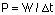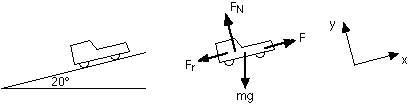## Power

10-18-99

Section 6.10

### Power

Being able to do work is not just what's important; how fast you can do work is also an important factor. Power is the measure of how fast work is done. Computers have more calculating power than we do; a sports car generally has a more powerful engine than an economy car. Power is the rate at which work is done and the rate at which energy is used. The unit for power is the watt (W).An interesting calculation is the average power output of a human being. This can be determined from the amount of energy we consume in a day in the way of food. Most of us take in something like 2500 "calories" in a day, although what we call calories is really a kilocalorie; assuming we use up all this energy in a day (a reasonable assumption considering we'll have to eat tomorrow, too) we can use this as our energy output per day.

First, take the 2.5 x 106 cal and convert to Joules, using the conversion factor 4.18 J / cal. This gives roughly 1 x 107 J. Figuring out our average power output, we simply divide the energy by the number of seconds in a day, 86400, which gives a bit more than 100 W. In other words, on the average, we're just a little brighter than your average light bulb.

### Calculating power from speed

Power is work over time, and work is force multiplied by distance. Power can be written as:

Power : P = F s / t (F is the force in the direction of s, the displacement)

Displacement over time is velocity, so power can also be written in this form:

Power : P = F v (F is the force in the direction of the velocity)

Here's an example of when you might use this. Let's say you're riding your bicycle on a level road at a constant speed of 10 m/s. You're riding into a headwind, and you're burning up energy at the rate of 500 J/s. If you assume that 80% of this energy is going to overcome air resistance, how much force is the air exerting on you?

The power used to overcome air resistance is 80% of 500 W, which is 400 W. Assuming there aren't any other forces acting against you, then dividing this by your speed should give you the force the air exerts on you. This works out to 40 N.

### Example - A car climbing a hill

A car with a mass of 900 kg climbs a 20° incline at a steady speed of 60 km/hr. If the total resistance forces acting on the car add to 500 N, what is the power output of the car in watts? In horsepower?Note that the gravitational force is the only force which needs to be split into components. mg sin20° acts down the slope; mg cos20° acts into the slope. Fr represents the resistance forces.

A good place to start here is with the free-body diagram. The power output by the car's engine goes into the force directed up the slope. This force is actually static friction exerted on the drive wheels by the road - the road exerts this force because the engine causes the drive wheels to rotate.

The velocity is constant, so the forces must balance. Applying Newton's second law in the x-direction gives:

F - Fr - mg sin20° = 0

The force up the slope is then F =Fr + mg sin20° = 500 + 3017 = 3517 N

Converting the car's speed to m/s gives 16.67 m/s. The power output can then be found from
P = Fv = (3517) (16.67) = 58620 W.

This can be converted to horsepower, using the conversion 746 W = 1 hp. This gives a power output of 78.6 hp.

Most cars have engines with power outputs of about 100 hp, so this is a reasonable value (and there's nothing in the question to say that this has to be the maximum power output of the car).